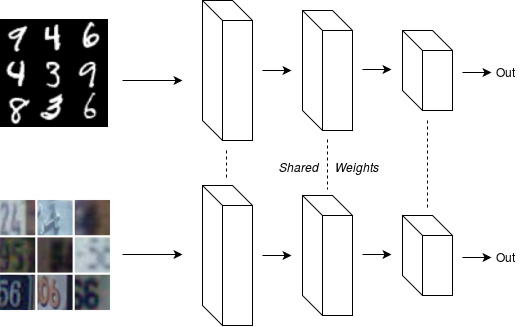# How to implement two networks with shared weights and with separate Batch Normalizations?

Hello,

I am working on a similar network similar to shared weight networks:This image is obtained from the following blog post, in which the author mentioned implementing separate batch normalizations for each network:

Any ideas on how to implement that in PyTorch please?

3 Likes

Maybe you can pass an additional input to the `forward` function indicating the image type and decide which batch norm to use, a simple example code:

``````import torch.nn as nn

class Model(nn.Module):
def __init__(self):
super(Model, self).__init__()
self.conv1 = nn.Conv2d(...)
self.conv2 = nn.Conv2d(...)
self.conv3 = nn.Conv2d(...)
self.bn1 = nn.BatchNorm2d(...)
self.bn2 = nn.BatchNorm2d(...)

def forward(x, data_type):
x = self.conv1(x)
x = self.conv2(x)
x = self.conv3(x)
if data_type == 'type1':
x = self.bn1(x)
elif data_type == 'type2':
x = self.bn2(x)

return x
``````
2 Likes

Thanks, @allenye0119!
I can see the idea now, but I don’t see how to use the modified `forward()` function. The training code at each epoch looks like this:

``````    optimizer.zero_grad()
output = model(data)
loss = criterion(output, target)
loss.backward()
optimizer.step()
``````

So I guess I have to modify `Model()` so that it takes an additional argument: `output = model(data, data_type)`? Thanks again for your help.

You don’t have to modify anything other than the `forward` function.
Just do `output = model(data, data_type)`.

1 Like

Oh, cool!
Great help. Thank you very much, @allenye0119!1 Like

Hi @allenye0119. I tried your idea on a more complex model and it is not easy at allFor example the following ResNet18:

``````class BasicBlock(nn.Module):
expansion = 1

def __init__(self, in_planes, planes, stride=1):
super(BasicBlock, self).__init__()
self.conv1 = conv3x3(in_planes, planes, stride)
self.bn1 = nn.BatchNorm2d(planes)
self.conv2 = conv3x3(planes, planes)
self.bn2 = nn.BatchNorm2d(planes)

self.shortcut = nn.Sequential()
if stride != 1 or in_planes != self.expansion*planes:
self.shortcut = nn.Sequential(
nn.Conv2d(in_planes, self.expansion*planes, kernel_size=1, stride=stride, bias=False),
nn.BatchNorm2d(self.expansion*planes)
)

def forward(self, x):
out = F.relu(self.bn1(self.conv1(x)))
out = self.bn2(self.conv2(out))
out += self.shortcut(x)
out = F.relu(out)
return out

class ResNet(nn.Module):
def __init__(self, block, num_blocks, num_classes=10):
super(ResNet, self).__init__()
self.in_planes = 64

self.conv1 = conv3x3(3,64)
self.bn1 = nn.BatchNorm2d(64)
self.layer1 = self._make_layer(block, 64, num_blocks, stride=1)
self.layer2 = self._make_layer(block, 128, num_blocks, stride=2)
self.layer3 = self._make_layer(block, 256, num_blocks, stride=2)
self.layer4 = self._make_layer(block, 512, num_blocks, stride=2)
self.linear = nn.Linear(512*block.expansion, num_classes)

def _make_layer(self, block, planes, num_blocks, stride):
strides = [stride] + *(num_blocks-1)
layers = []
for stride in strides:
layers.append(block(self.in_planes, planes, stride))
self.in_planes = planes * block.expansion
return nn.Sequential(*layers)

def forward(self, x):
out = F.relu(self.bn1(self.conv1(x)))
out = self.layer1(out)
out = self.layer2(out)
out = self.layer3(out)
out = self.layer4(out)
out = F.avg_pool2d(out, 4)
out = out.view(out.size(0), -1)
out = self.linear(out)
return out

def ResNet18():
return ResNet(BasicBlock, [2,2,2,2])
``````

I guess I have to be able to do `self.shortcut(x, data_type)` and `self.layer1(out, data_type)` but I have no idea how to do that. I can unroll the blocks and the layers to get the 18 layers explicitly and then modify the forward function for each layer, but I don’t think it is a reasonable solution.

Do you have any ideas on that? Thank you so much again!

I think the main difficulty stems from the use of `nn.Sequential`, which only takes in a single argument `input`. So it’s not possible to do branching in `nn.Sequential` when additional info is required (in this case, the data type). Which means that we need to replace all `nn.Sequential`.

For `BasicBlock`:

``````class BasicBlock(nn.Module):
expansion = 1

def __init__(self, in_planes, planes, stride=1):
super(BasicBlock, self).__init__()
self.conv1 = conv3x3(in_planes, planes, stride)
self.bn1 = nn.BatchNorm2d(planes)
self.conv2 = conv3x3(planes, planes)
self.bn2 = nn.BatchNorm2d(planes)

# self.shortcut = nn.Sequential()
# if stride != 1 or in_planes != self.expansion*planes:
#     self.shortcut = nn.Sequential(
#         nn.Conv2d(in_planes, self.expansion*planes, kernel_size=1, stride=stride, bias=False),
#         nn.BatchNorm2d(self.expansion*planes)
#     )
if stride != 1 or in_planes != self.expansion*planes:
self.identity_shortcut = False
self.shortcut_conv = nn.Conv2d(in_planes, self.expansion*planes, kernel_size=1, stride=stride, bias=False)
self.shortcut_bn1 = nn.BatchNorm2d(self.expansion*planes)
self.shortcut_bn2 = nn.BatchNorm2d(self.expansion*planes)
else:
self.identity_shortcut = True

def forward(self, x, data_type):
out = F.relu(self.bn1(self.conv1(x)))
out = self.bn2(self.conv2(out))

# out += self.shortcut(x)
if not self.identity_shortcut:
x = self.shortcut_conv(x)
if data_type == 'type1':
x = self.sortcut_bn1(x)
elif data_type == 'type2':
x = self.sortcut_bn2(x)
out += x

out = F.relu(out)
return out
``````

For `ResNet._make_layer`:

``````def _make_layer(self, block, planes, num_blocks, stride):
strides = [stride] + *(num_blocks-1)
layers = []
for stride in strides:
layers.append(block(self.in_planes, planes, stride))
self.in_planes = planes * block.expansion
return nn.ModuleList(layers)
``````

and `ResNet.forward`:

``````def forward(self, x, data_type):
out = F.relu(self.bn1(self.conv1(x)))
# out = self.layer1(out)
# out = self.layer2(out)
# out = self.layer3(out)
# out = self.layer4(out)
for layer in self.layer1:
out = layer(out, data_type)
for layer in self.layer2:
out = layer(out, data_type)
for layer in self.layer3:
out = layer(out, data_type)
for layer in self.layer4:
out = layer(out, data_type)
out = F.avg_pool2d(out, 4)
out = out.view(out.size(0), -1)
out = self.linear(out)
return out
``````

This is only a quick fix, I’m sure the code can be made more elegant.

1 Like

You’re awesome, @allenye0119!That’s already much more elegant than I expected! Thanks again!

Hi, I also want to implement a split batch norm layer and I can decide to use which in different forward steps. I want to do something like these:

``````output1=model(data1)
model.switch("bn2")
output2=model(data2)
model.switch("bn1")
output3=model(data3)
...
``````

How can I implement such feature?